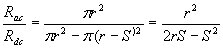# Skin Effect Calculator

Because of skin effect ac current tends to flow on the outside of a connector. On a wire the current density looks like a hollow tube. Since the inside isn't used for AC current flow, it makes sense to eliminate as much of the hollow part as possible.

Skin depth is defined as the distance below the surface where the current density has fallen to 1/e or 37% of its value at the surface.

For copper at 70C:

S= 2837/sqrt(f)

Where f is frequency in Hertz, and S is the skin depth in mils.

where:

 Frequency: f (KHz) Skin Depth: S (mils) Wire gauge such that Rac=Rdc AWG

A useful ratio is the AC resistance to the DC resistance of the conductor. Resistance is inversely proportional to the cross sectional area of the conductor. For a round wire the ratio is expressed as:As a rule of thumb, in selecting the proper wire gauge, we want the DC resistance to equal the AC resistance. We can determine when the AC resistance is equal to the DC resistance by setting this ratio to one.Clearly, when r=S, or the diameter=2S, the two resistances are equal. For this case it is easy to see that the wire is being completely utilized throughout it's cross section for AC current flow. In other words, the conductor has been sized so that there is no wasted inner core, where AC current doesn't flow.

[Plant Database], [Soil Moisture Sensor] [Water Level Sensor] [Soil Moisture Meter]